## 简介

1. Wide & Deep模型，包含前馈神经网络embedding部分以及以及线性模型特征转换，在广义推荐系统当中的应用
2. Wide & Deep模型在Google Play场景下的实现与评估，Google Play是一个拥有超过10亿日活和100w App的移动App商店

## 推荐系统概述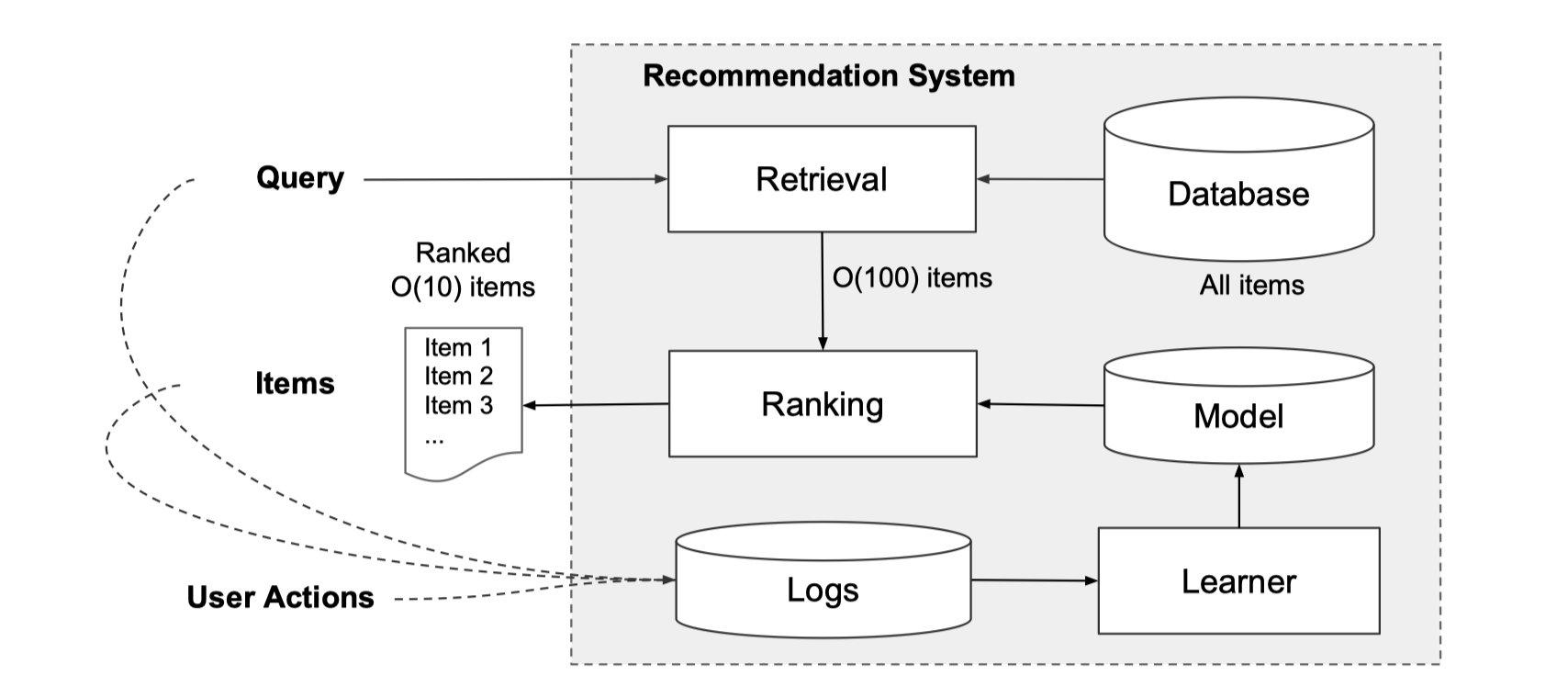## Wide & Deep原理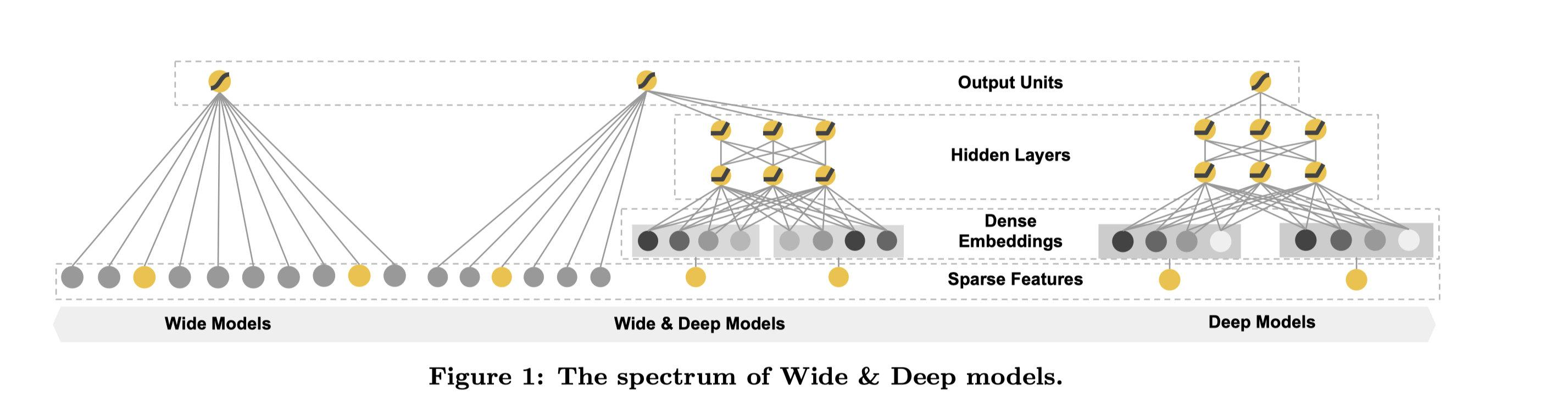### Wide部分

Wide部分其实就是一个泛化的形如 $y = w^Tx + b$的线性模型，就如上图左边部分所展示的一样。y是我们要预测的结果，x是特征，它是一个d维的向量 $x=[x_1,x_2,\cdots,x_d]$。这里的d是特征的数量。同样w也是一个d维的权重向量 $w=[w_1,w_2,\cdots,w_d]$，b呢则是偏移量。这些我们在之前线性回归的模型当中曾经都介绍过，大家应该也都不陌生。

$\phi_k(x)=\Pi_{i=1}^dx_i^{c_{ki}}\quad c_{ki}\in\{0, 1\}$

### Deep部分

Deep部分是一个前馈神经网络，也就是上图当中的右侧部分。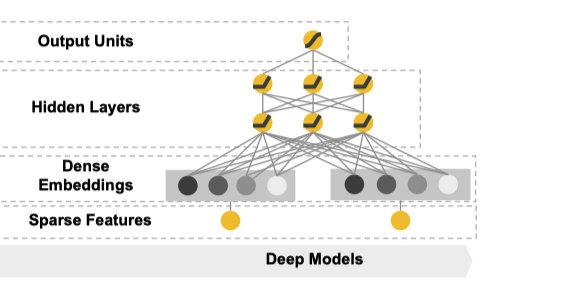### Wide & Deep合并

Wide部分和Deep部分都有了之后，通过加权的方式合并在一起。这也就是上图当中的中间部分。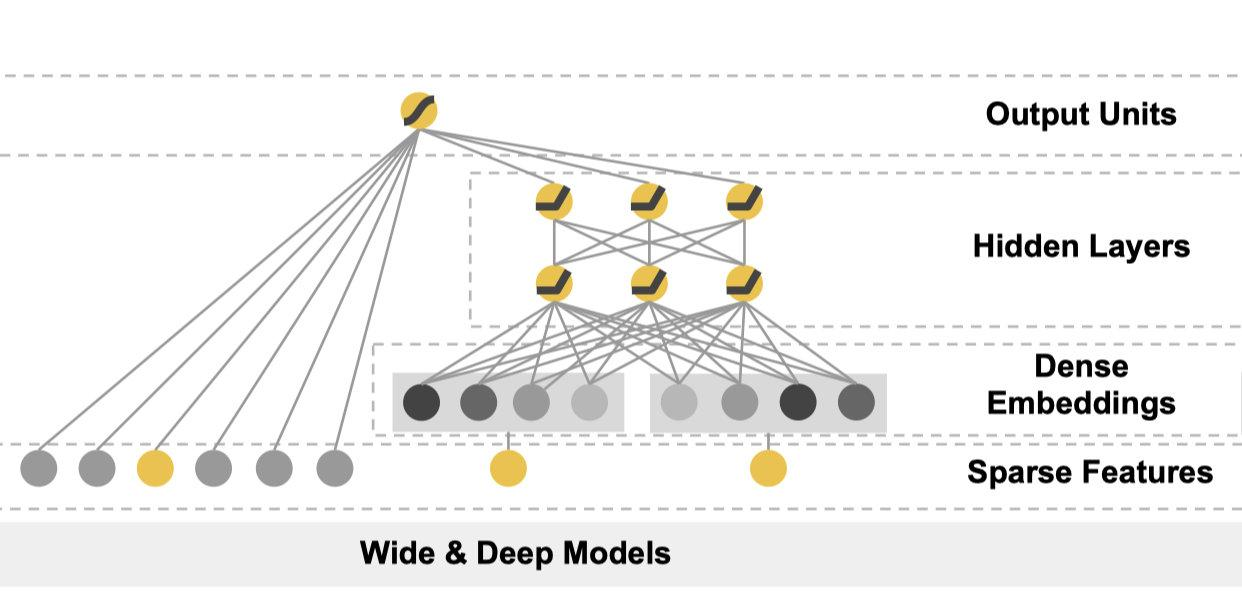## 系统实现

app推荐的数据流包含了三个部分：数据生产、模型训练以及模型服务。用一张图来展示大概是这样的：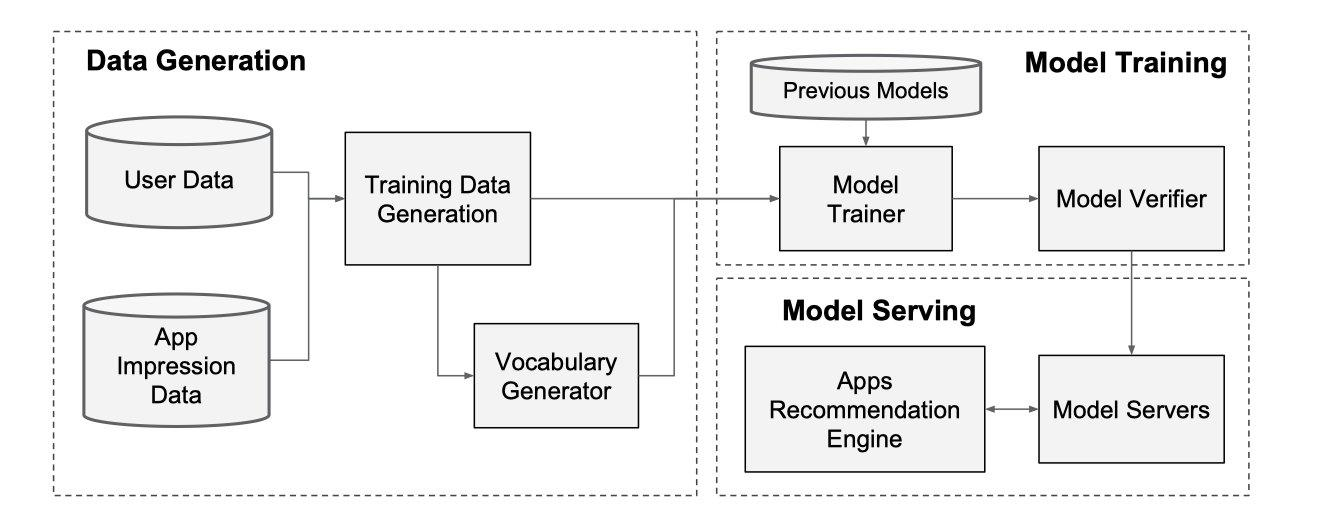### 模型训练

paper当中提供了一张模型的结构图：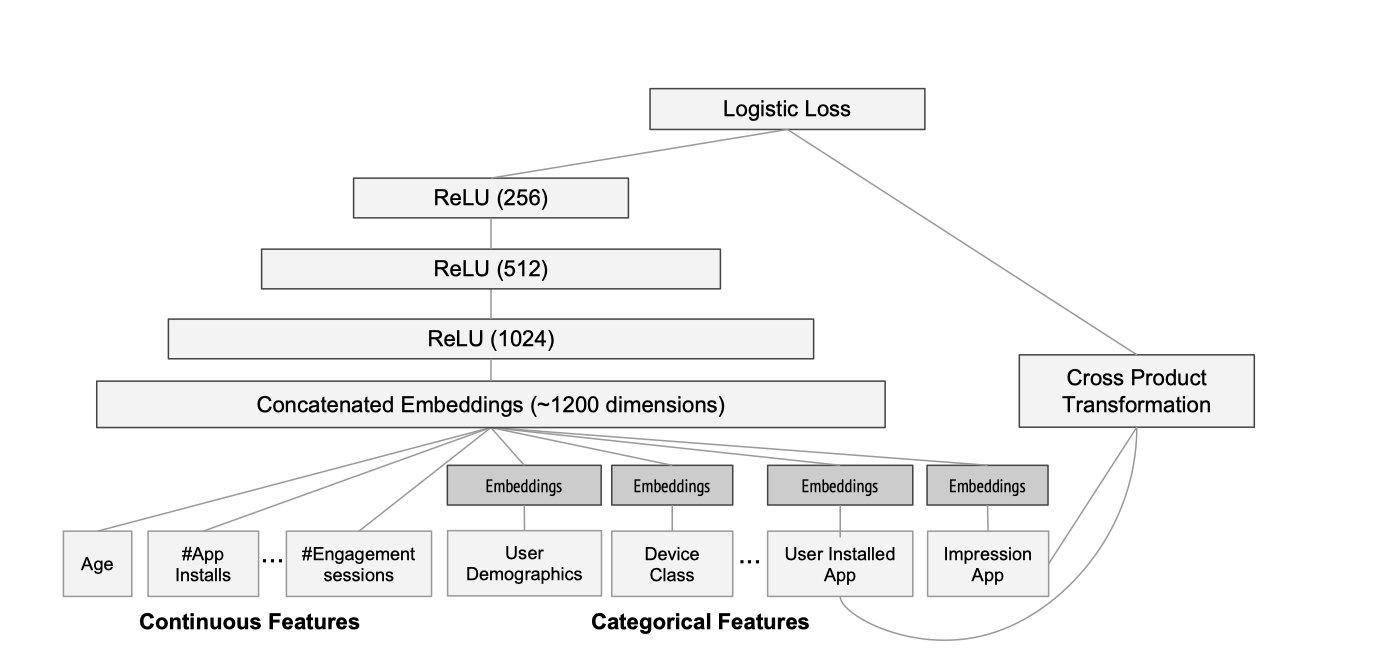## 模型结果

### App 获取量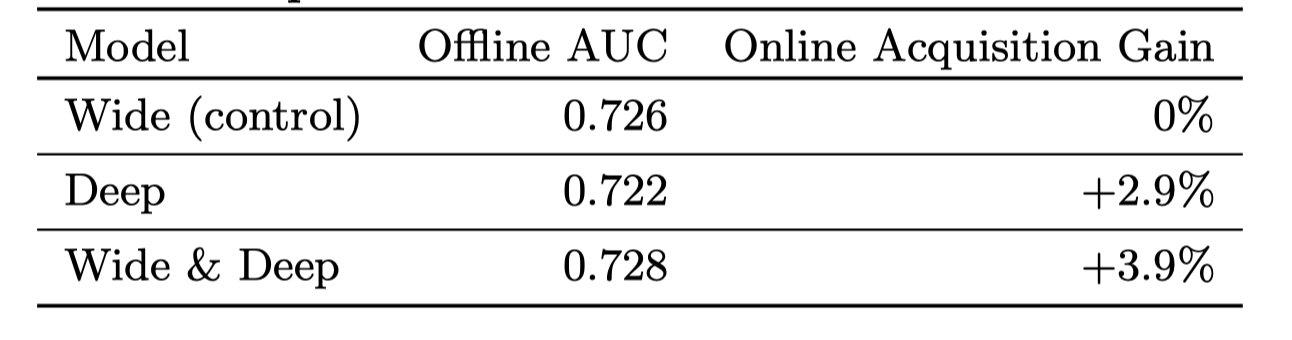Wide & Deep模型不仅AUC更高，并且线上APP的获取量也提升了3.9%。

### 服务性能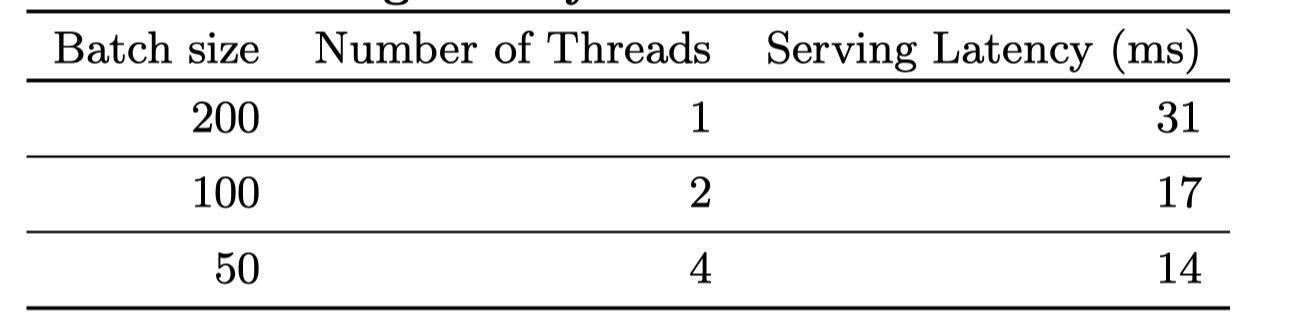## 代码实现

import torch
from torch import nn

class WideAndDeep(nn.Module):
def __init__(self, dense_dim=13, site_category_dim=24, app_category_dim=32):
super(WideAndDeep, self).__init__()
# 线性部分
self.logistic = nn.Linear(19, 1, bias=True)
# embedding部分
self.site_emb = nn.Embedding(site_category_dim, 6)
self.app_emb = nn.Embedding(app_category_dim, 6)
# 融合部分
self.fusion_layer = nn.Linear(12, 6)

def forward(self, x):
site = self.site_emb(x[:, -2].long())
app = self.app_emb(x[:, -1].long())
emb = self.fusion_layer(torch.cat((site, app), dim=1))
return torch.sigmoid(self.logistic(torch.cat((emb, x[:, :-2]), dim=1)))Python

466

#### 相关文章推荐